Next: Xia's Reduction Algorithm Up: Complexity Previous: Endomorphism Graphs

## Fixed Point Free Automorphisms

The problem of deciding whether a finite ordered set has a fixed point free automorphism was found by Williamson to be NP-complete (cf. , Fact 5.1 or Theorem 2.14 here), i.e.,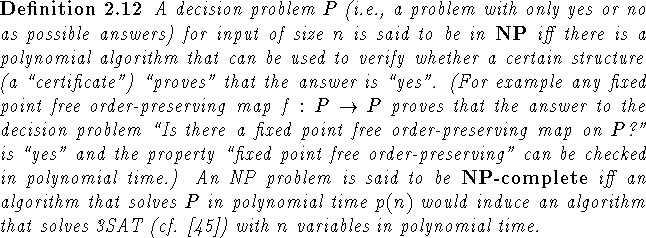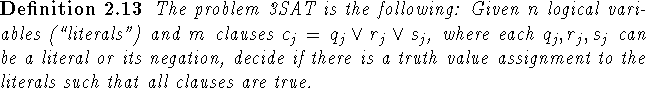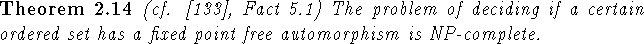We will present two proofs of this theorem here. One by Goddard that reduces the problem to a known NP-complete problem, namely the determination if a given graph has a fixed point free automorphism (cf. ). The other is Williamson's original adaptation of Lubiw's proof in , which reduces the problem to 3SAT. This proof will be sketched only. For the first proof we need: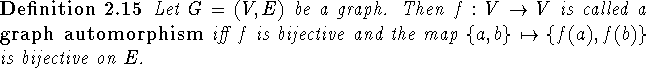Proof 1 of Theorem 2.14: (T. Goddard, private communication, also cf. ) By  it is NP-complete to decide if a given graph has a fixed point free automorphism. We will show that any algorithm that decides if an arbitrary ordered set P has a fixed point free automorphism in q(|P|) steps also decides if an arbitrary graph G=(V,E) has a fixed point free automorphism in q'(|V|) steps, where q,q' are polynomials.
Let G=(V,E) be a graph. Order the set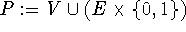via the order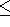defined as follows:

1.is reflexive,
2. No two vertices of G are related,
3. No two elements of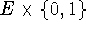are related,
4. If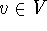and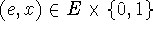, then v<(e,x) iff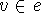.
Clearly |P| is bounded by a polynomial function of |V|. Moreover P has a fixed point free automorphism iff G does: If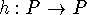is a fixed point free automorphism, then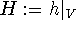is a fixed point free automorphism of G. Conversely, if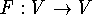is a fixed point free automorphism of G, then let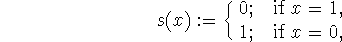and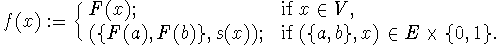f is a fixed point free automorphism of P. \

Thus we even know that the problem to decide if an ordered set of height 1 has a fixed point free automorphism is NP-complete (this is also noted as Fact 5.2 in ). This is interesting in the light of Theorem 3.21 and Corollary 3.24, which show that there is an efficient algorithm to detect if an ordered set of height 1 has a fixed point free order-preserving self map. Our second proof is a reduction to 3SAT itself.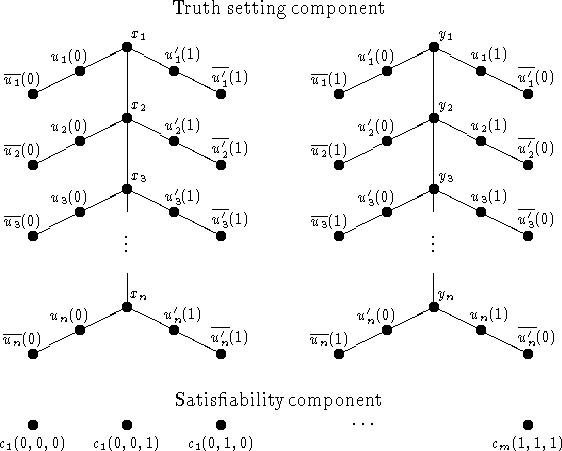Figure 2: The ordered set used in the second proof of Theorem 2.14: Consider an instance of 3SAT with m distinct clauses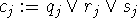, each of which contains exactly 3 distinct literals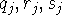out of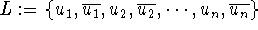. The satisfiabiliy component is an antichain of points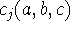, where abc counts from zero to 7 in binary. The truth setting component has comparabilities as indicated. Finally (not indicated in the figure) the upper covers ofare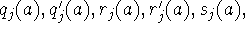and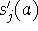.

Proof 2 of Theorem 2.14: (cf. , Fact 5.1; we present a sketch) For a given instance C of 3SAT which we can without loss of generality choose to have m distinct clauses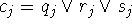each of which has 3 distinct literalsout ofwe construct the ordered set P as indicated in Figure 2. Note that eachhas a unique set of 6 upper covers. For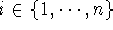, let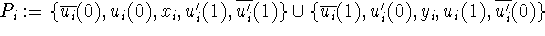. Then it is easy to see that every automorphism of P must fix the satisfiability component and every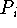. Eachhas exactly four fixed point free automorphisms, of which only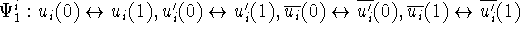and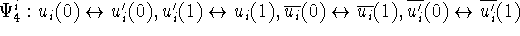(we remain consistent with the notation in  here) can possibly extend to an automorphism of P (the other two map sets that have a lower bound in the satisfiability component to sets that do not have lower bounds).
To show that P has a fixed point free automorphism iff there is a satisfying assignment for C first assume that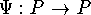is a fixed point free automorphism. If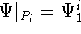, set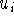TRUE. Otherwise we have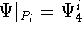, and we setFALSE. Now if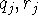and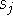were all false for some j, then the definitions of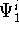and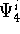indicate that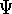must interchange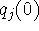and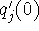,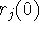and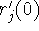, and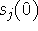and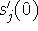. Since these are the upper covers of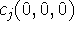we conclude thatis a fixed point of, a contradiction.
On the other hand if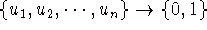is a satisfying assignment for C (with 0 standing for FALSE and 1 standing for TRUE as usual), we defineas follows: Set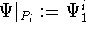ifis true (1), set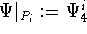ifis false (0) and set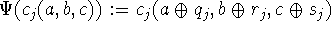, where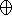denotes addition modulo 2. Since one ofis 1 (true),has no fixed points.trivially is an automorphism on the truth setting component. Since addition of a 1 or a 0 modulo 2 is injective,is injective and hence bijective on the satisfiability component. Finally one checks thatis order-preserving. \

Using the characterization in Remark 2.11 we can also record some known results as easy consequences: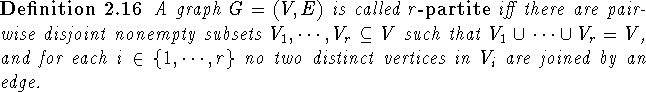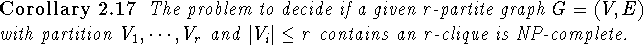Proof: If there was an algorithm that decides if a given r-partite graph G=(V,E) with partition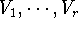and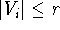contains an r-clique in q(|V|) steps, then by Remark 2.11 there would be an algorithm that determines if the fixed point free automorphism graph of a given ordered set P has a |P|-clique in q'(|P|) steps. Thus we could determine if a given ordered set P has a fixed point free automorphism in q''(|P|) steps (q,q',q'' all polynomials).\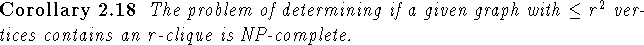Proof: If there was an algorithm that determines if a given graph G=(V,E) with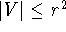has an r-clique in q(|V|) steps, then this algorithm would decide if a given r-partite graph G=(V,E) with partitionandhas an r-clique in q(|V|) steps. \Next: Xia's Reduction Algorithm Up: Complexity Previous: Endomorphism Graphs

Bernd.S.W.Schroder